Main Content

# lazysnapping

Segment image into foreground and background using graph-based segmentation

## Syntax

``BW = lazysnapping(A,L,foremask,backmask)``
``BW = lazysnapping(A,L,foreind,backind)``
``BW = lazysnapping(___,Name,Value)``

## Description

example

````BW = lazysnapping(A,L,foremask,backmask)` segments the image `A` into foreground and background regions using lazy snapping. The label matrix `L` specifies the subregions of the image. `foremask` and `backmask` are masks designating pixels in the image as foreground and background, respectively.```

example

````BW = lazysnapping(A,L,foreind,backind)` segments the image `A` into foreground and background regions. `foreind` and `backind` specify the linear indices of the pixels in the image marked as foreground and background, respectively.```
````BW = lazysnapping(___,Name,Value)` segments the image or volume using name-value pairs to control aspects of the segmentation.```

## Examples

collapse all

Read and display an image.

```RGB = imread('peppers.png'); imshow(RGB)```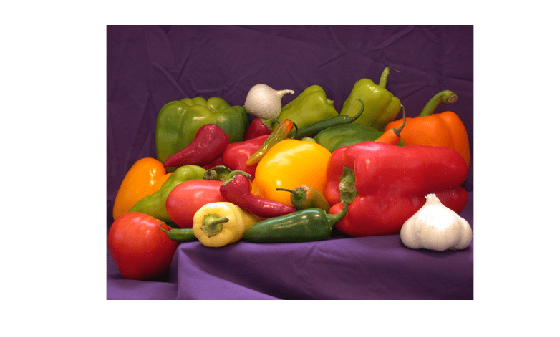Create a label matrix.

`L = superpixels(RGB,500);`

Specify a rectangular ROI within the foreground by using the `drawrectangle` function. The 'Position' name-value pair argument specifies the upper left coordinates, width, and height of the ROI as the 4-element vector `[xmin,ymin,width,height]`. If you want to draw the rectangle interactively, then omit the 'Position' name-value pair argument.

`f = drawrectangle(gca,'Position',[100 128 350 150],'Color','g');`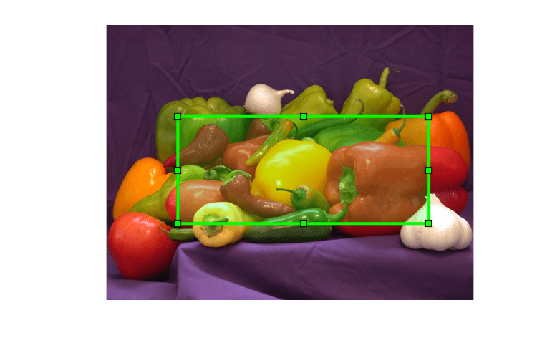Create a mask that contains the foreground pixels.

`foreground = createMask(f,RGB);`

Specify background ROIs. To improve the segmentation accuracy, this example specifies two rectangular ROIs in different areas of the background.

```b1 = drawrectangle(gca,'Position',[130 30 40 30],'Color','r'); b2 = drawrectangle(gca,'Position',[6 368 500 10],'Color','r');```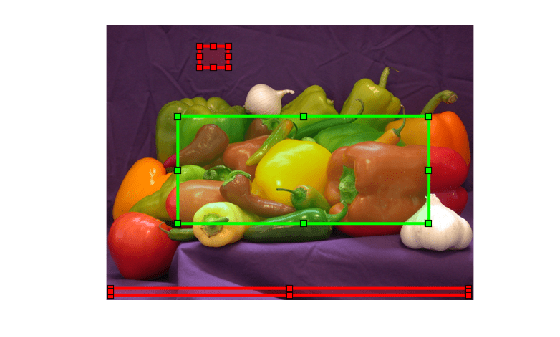Create a mask that contains the background pixels. This mask is the union of the two background ROIs.

`background = createMask(b1,RGB) + createMask(b2,RGB);`

Perform lazy snapping.

`BW = lazysnapping(RGB,L,foreground,background);`

Visualize the result of the segmentation by highlighting the foreground in green.

`imshow(labeloverlay(RGB,BW,'Colormap',[0 1 0]))`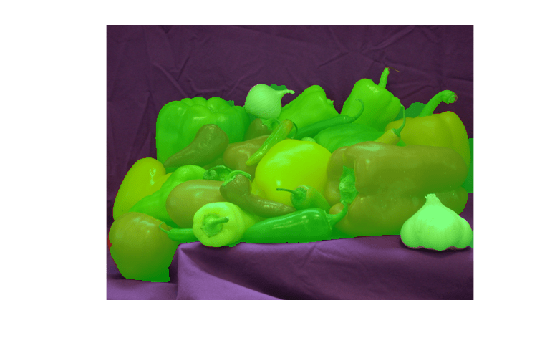Create a masked image in which the background is black.

```maskedImage = RGB; maskedImage(repmat(~BW,[1 1 3])) = 0; imshow(maskedImage)```Read and display an image.

```RGB = imread('peppers.png'); imshow(RGB)```Create a label matrix.

`L = superpixels(RGB,500);`

Specify the x- and y-coordinates of pixels in the foreground.

```foregroundX = [34 114 195 259 392 467 483]; foregroundY = [298 140 135 200 205 283 104];```

Convert the coordinates to linear indices. `sub2ind` takes (row, column) coordinates so specify the input arguments with the y-coordinates before the x-coordinates.

`foregroundInd = sub2ind(size(RGB),foregroundY,foregroundX);`

Specify the x- and y-coordinates of pixels in the background.

```backgroundX = [130 170]; backgroundY = [52 32];```

Convert the coordinates to linear indices.

`backgroundInd = sub2ind(size(RGB),backgroundY,backgroundX);`

Perform lazy snapping.

`BW = lazysnapping(RGB,L,foregroundInd,backgroundInd);`

Display the segmented mask. Foreground pixels are `true` and background pixels are `false`.

`imshow(BW)`Display the mask over the original image, highlighting foreground pixels in green.

`imshow(labeloverlay(RGB,BW,'Colormap',[0 1 0]))`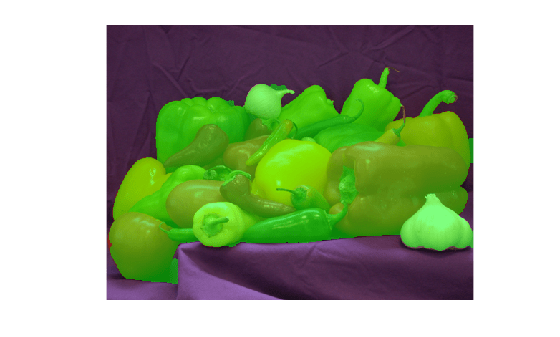Load 3-D volumetric image into the workspace.

```D = load('mri.mat'); V = squeeze(D.D); ```

Create a 2-D mask identifying initial foreground and background seed points.

``` seedLevel = 10; fseed = V(:,:,seedLevel) > 75; bseed = V(:,:,seedLevel) == 0; figure; imshow(fseed)```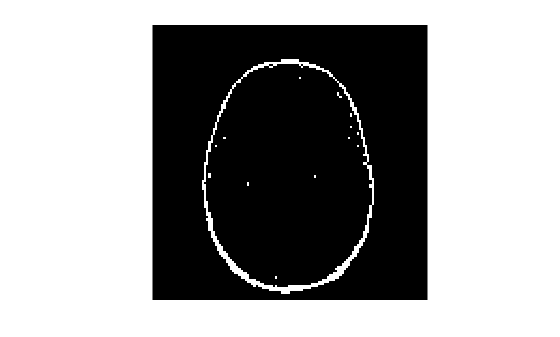``` figure; imshow(bseed)```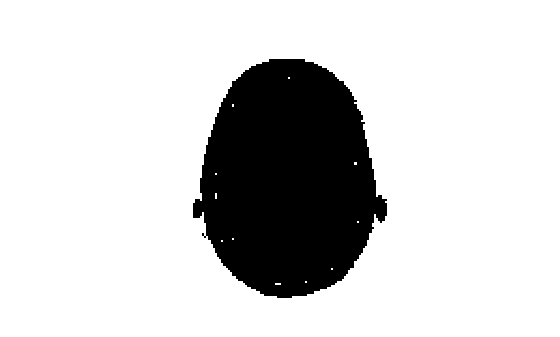Place seed points into empty 3-D mask.

```fmask = zeros(size(V)); bmask = fmask; fmask(:,:,seedLevel) = fseed; bmask(:,:,seedLevel) = bseed;```

Generate a 3-D label matrix.

` L = superpixels3(V,500);`

Segment the image into foreground and background using Lazy Snapping.

`bw = lazysnapping(V,L,fmask,bmask);`

Display the 3-D segmented image.

```figure; p = patch(isosurface(double(bw))); p.FaceColor = 'red'; p.EdgeColor = 'none'; daspect([1 1 27/128]); camlight; lighting phong```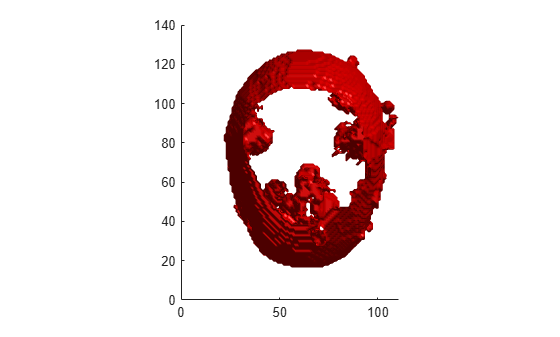## Input Arguments

collapse all

Image to segment, specified as a 2-D grayscale, truecolor, or multispectral image or a 3-D grayscale volume. For `double` and `single` images, `lazysnapping` assumes the range of the image to be [0, 1]. For `uint16`, `int16`, and `uint8` images, `lazysnapping` assumes the range to be the full range for the given data type. If the values do not match the expected range based on the data type, then scale the image to the expected range or adjust `EdgeWeightScaleFactor` to improve results.

Data Types: `single` | `double` | `int16` | `uint8` | `uint16`

Label matrix of the input image or volume, specified as numeric array. For 2-D grayscale images and 3-D grayscale volumes, the size of `L` must match the size of the input image `A`. For color images and multichannel images, `L` must be a 2-D array where the first two dimensions match the first two dimensions of the input image `A`.

Do not mark a given subregion of the label matrix as belonging to both the foreground mask and the background mask. If a region of the label matrix contains pixels belonging to both the foreground mask and background mask, `lazysnapping` segments the region as background.

Data Types: `single` | `double` | `int8` | `int16` | `int32` | `int64` | `uint8` | `uint16` | `uint32` | `uint64` | `logical`

Mask image that defines the foreground, specified as a logical array. For 2-D grayscale images and 3-D grayscale volumes, the size of `foremask` must match the size of the input image `A`. For color images and multichannel images, `foremask` must be a 2-D array where the first two dimensions match the first two dimensions of the input image `A`.

Data Types: `single` | `double` | `int8` | `int16` | `int32` | `int64` | `uint8` | `uint16` | `uint32` | `uint64` | `logical`

Mask image that defines the background, specified as a logical array. For 2-D grayscale images and 3-D grayscale volumes, the size of `backmask` must match the size of the input image `A`. For color images and multichannel images, `backmask` must be a 2-D array where the first two dimensions match the first two dimensions of the input image `A`.

Data Types: `single` | `double` | `int8` | `int16` | `int32` | `int64` | `uint8` | `uint16` | `uint32` | `uint64` | `logical`

Linear index of pixels in the label matrix, specified as a numeric vector.

Data Types: `single` | `double` | `int8` | `int16` | `int32` | `int64` | `uint8` | `uint16` | `uint32` | `uint64` | `logical`

Linear index of pixels that define the background, specified as a numeric vector.

Data Types: `single` | `double` | `int8` | `int16` | `int32` | `int64` | `uint8` | `uint16` | `uint32` | `uint64` | `logical`

### Name-Value Pair Arguments

Specify optional comma-separated pairs of `Name,Value` arguments. `Name` is the argument name and `Value` is the corresponding value. `Name` must appear inside quotes. You can specify several name and value pair arguments in any order as `Name1,Value1,...,NameN,ValueN`.

Example: `'Connectivity',6`

Connectivity of connected components, specified as the comma-separated pair consisting of `'Connectivity'` and one of the following: 4 or 8, for 2-D images, and 6, 18, or 26 for 3-D images (volumes).

Data Types: `single` | `double` | `int8` | `int16` | `int32` | `int64` | `uint8` | `uint16` | `uint32` | `uint64`

Scale factor for edge weights between the subregions of the label matrix, specified as the comma-separated pair consisting of `'EdgeWeightScaleFactor'` and a positive number. Typical values range from [10, 1000]. Increasing this value increases the likelihood that `lazysnapping` labels neighboring subregions together as either foreground or background.

Data Types: `single` | `double` | `int8` | `int16` | `int32` | `int64` | `uint8` | `uint16` | `uint32` | `uint64`

## Output Arguments

collapse all

Segmented image, returned as a logical array of the same size as the label matrix, `L`.

Data Types: `logical`

## Tips

• The lazy snapping algorithm developed by Li et al. clusters foreground and background values using the K-means method. This implementation of the lazy snapping algorithm does not cluster similar foreground or background pixels. To improve performance, reduce the number of pixels with similar values that are identified as foreground or background.

• To obtain masks `foremask` or `backmask` interactively, you can draw an ROI on the image then create a mask from the ROI by using the `createMask` function. For more information, see ROI Creation Overview.

• To obtain pixel indices `foreind` or `backind` interactively, you can draw a `Polyline` ROI object by using the `drawpolyline` function. Get the x- and y-coordinates of the vertices from the `Position` property of the `Polyline`. Finally, convert the coordinates to linear indices by using the `sub2ind` function. Note that the `sub2ind` function uses (row, column) coordinates instead of (x, y) coordinates.

 Y. Li, S. Jian, C. Tang, H. Shum, Lazy Snapping In Proceedings from the 31st International Conference on Computer Graphics and Interactive Techniques, 2004.

Download ebook